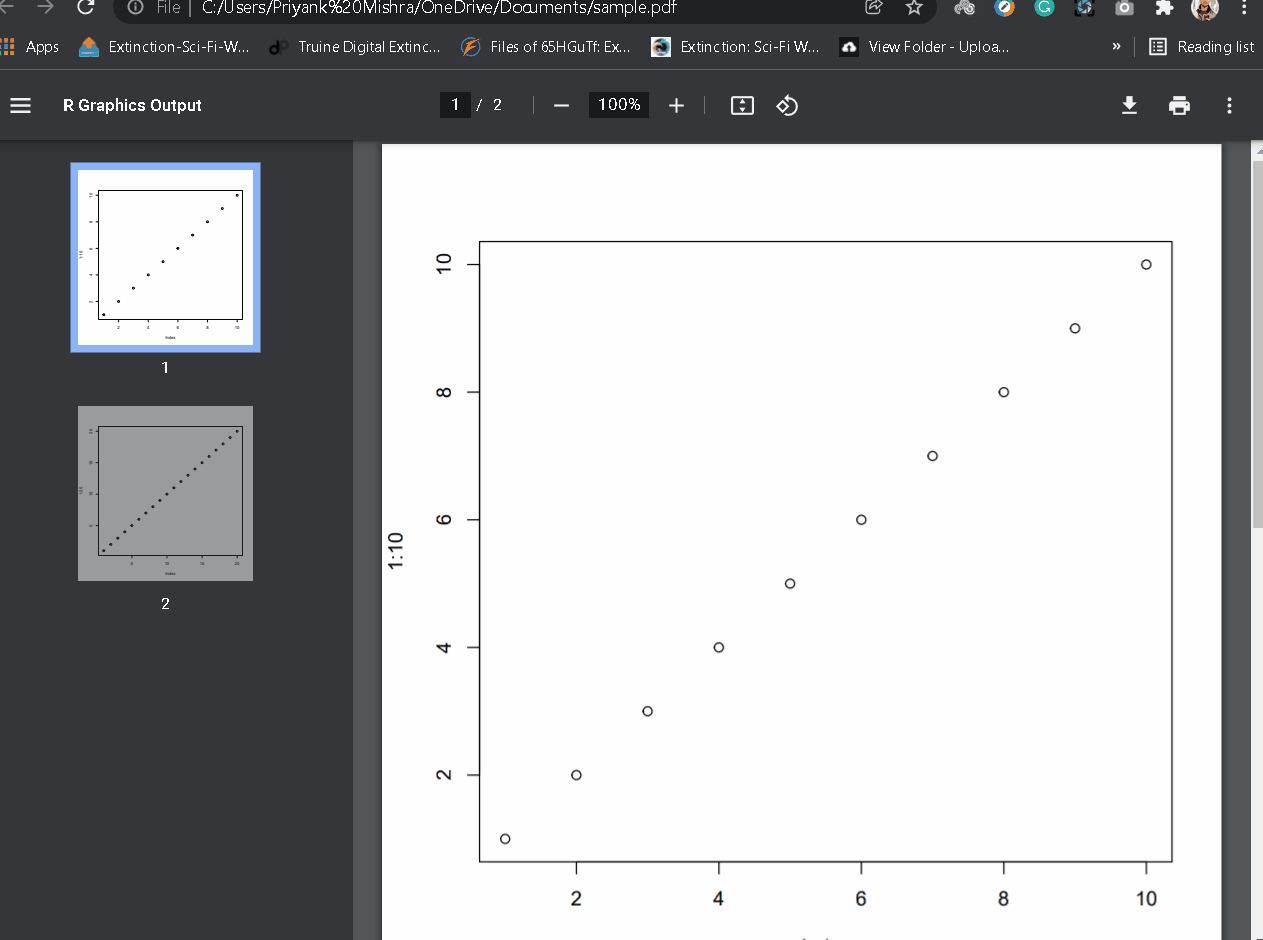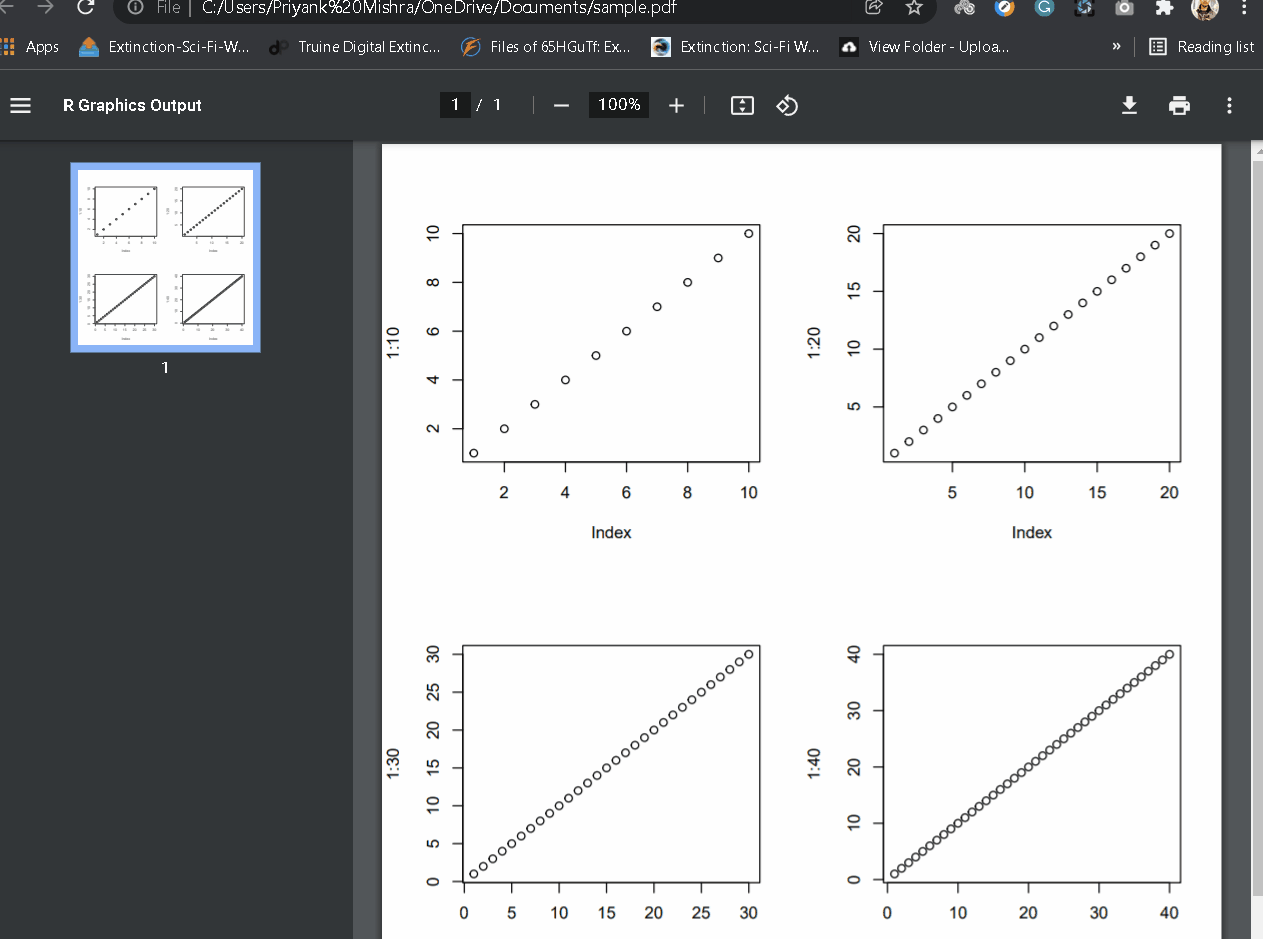GeeksforGeeks App
Open AppBrowser
Continue

# How to Export Multiple Plots to PDF in R?

In this article, we will learn how to export multiple plots to a PDF in the R Programming Language.

## Save Multiple plots on different pages on PDF file:

To save multiple plots in pdf we use the pdf() function to create and open a pdf file in the R Language.

After this, whatever we do in the R console gets saved in the pdf file opened. The pdf() function takes the file parameter which contains the name of the pdf along with the relative path to the working directory.

Syntax:

pdf( file )

where,

• file: determines the name of pdf along with the relative address to the working directory.

Example:

Here, we have saved multiple plots to the different pages in the pdf.

## R

 `# Open pdf file``pdf``(file= ``"sample.pdf"` `)`` ` `# draw plots``plot``(1:10)``plot``(1:20)`

Output:## Save Multiple Plots to Same Page in PDF:

To save multiple plots to the same page in the PDF file, we use the par() function to create a grid and then add plots to the grid. In this way, all the plots are saved on the same page of the pdf file. We use the mfrow argument to the par() function to create the desired grid.

Syntax:

par( mfrow )

where,

• mfrow: determines the vector that contains the number of rows and columns for the grid.

Example:

Here, is a program to save 4 plots in a 2X2 grid on the same page of a pdf file.

## R

 `# Open pdf file``pdf``(file= ``"sample.pdf"` `)`` ` `# create a 2X2 grid``par``( mfrow= ``c``(2,2) )`` ` `# draw plots``plot``(1:10)``plot``(1:20)``plot``(1:30)``plot``(1:40)`

Output:My Personal Notes arrow_drop_up